Get Free NCERT Solutions for Class 10 Maths Chapter 8 Ex 8.4 Introduction to Trigonometry Class 10 Maths NCERT Solutions are extremely helpful while doing homework. Exercise 8.4 Class 10 Maths NCERT Solutions were prepared by Experienced LearnCBSE.in Teachers. Detailed answers of all the questions in Chapter 8 Maths Class 10 Introduction to Trigonometry Exercise 8.4 Provided in NCERT Textbook.

Topics and Sub Topics in Class 10 Maths Chapter 8 Introduction to Trigonometry:

 Section Name Topic Name 8 Introduction to Trigonometry 8.1 Introduction 8.2 Trigonometric Ratios 8.3 Trigonometric Ratios Of Some Specific Angles 8.4 Trigonometric Ratios Of Complementary Angles 8.5 Trigonometric Identities 8.6 Summary

You can also download the free PDF of Chapter 8 Ex 8.4 Coordinate Geometry NCERT Solutions or save the solution images and take the print out to keep it handy for your exam preparation.

 Board CBSE Textbook NCERT Class Class 10 Subject Maths Chapter Chapter 8 Chapter Name Introduction to Trigonometry Exercise Ex 8.4 Number of Questions Solved 5 Category NCERT Solutions

## NCERT Solutions For Class 10 Maths Chapter 8 Introduction to Trigonometry Ex 8.4

NCERT Solutions for Class 10 Maths Chapter 8 Introduction to Trigonometry Ex 8.4 are part of NCERT Solutions for Class 10 Maths. Here we have given NCERT Solutions for Class 10 Maths Chapter 8 Introduction to Trigonometry Exercise 8.4

Ex 8.4 Class 10 Maths Question 1.

Express the trigonometric ratios sin A, sec A and tan A in terms of cot A.Solution: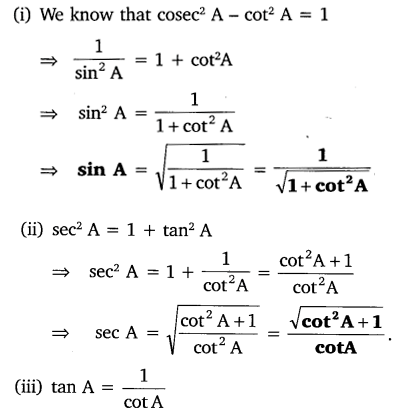Ex 8.4 Class 10 Maths Question 2.
Write all the other trigonometric ratios of ∠A in terms of sec A.
Solution:Download NCERT Solutions For Class 10 Maths Chapter 8 Introduction to Trigonometry PDF

Ex 8.4 Class 10 Maths Question 3.
Evaluate: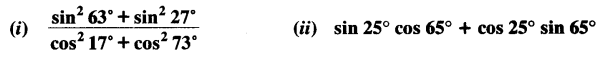Solution: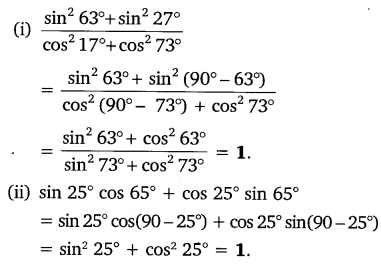Ex 8.4 Class 10 Maths Question 4.
Choose the correct option. Justify your choice.
(i) 9 sec² A – 9 tan² A = ……
(A) 1
(B) 9
(C) 8
(D) 0

(ii) (1 + tan θ + sec θ) (1 + cot θ – cosec θ) = ………..
(A) 0
(B) 1
(C) 2
(D) -1

(iii) (sec A + tan A) (1 – sin A) = ………….
(A) sec A
(B) sin A
(C) cosec A
(D) cos A

(iv) $$\frac { 1+{ tan }^{ 2 }A }{ 1+cot^{ 2 }A }$$ = ………..
(A) sec² A
(B) -1
(C) cot² A
(D) tan² A
Solution: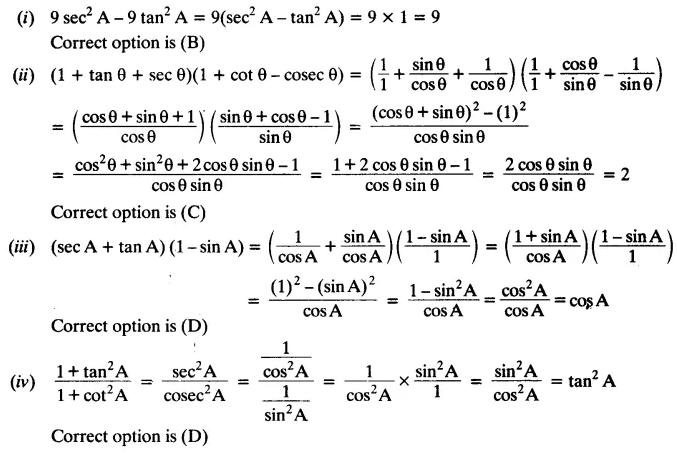Ex 8.4 Class 10 Maths Question 5.
Prove the following identities, where the angles involved are acute angles for which the expressions are defined.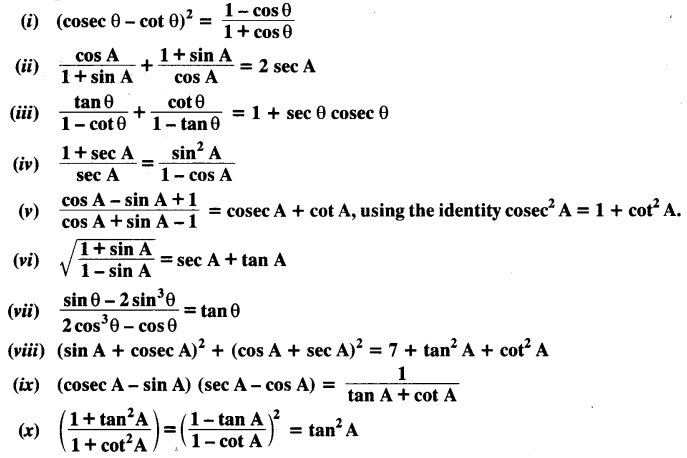Solution: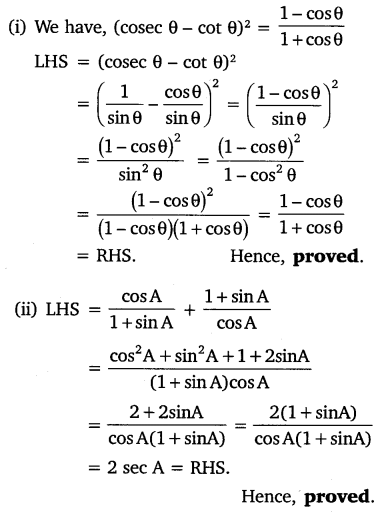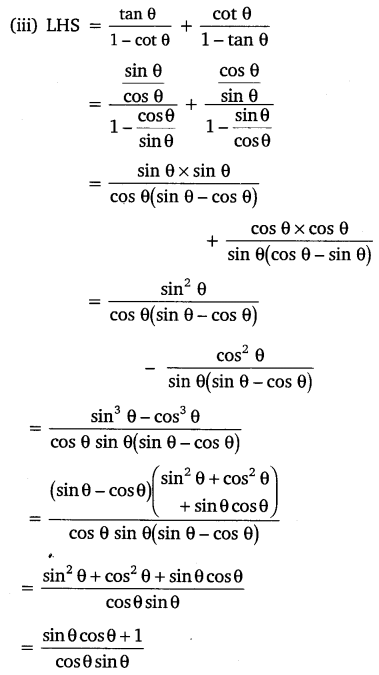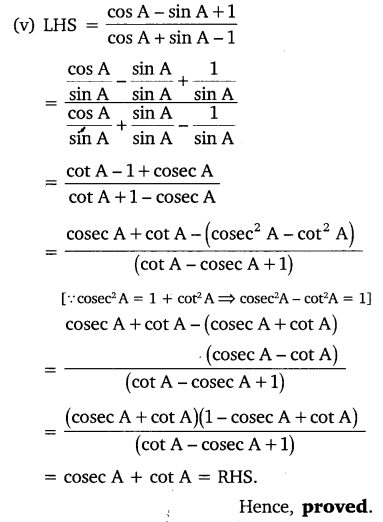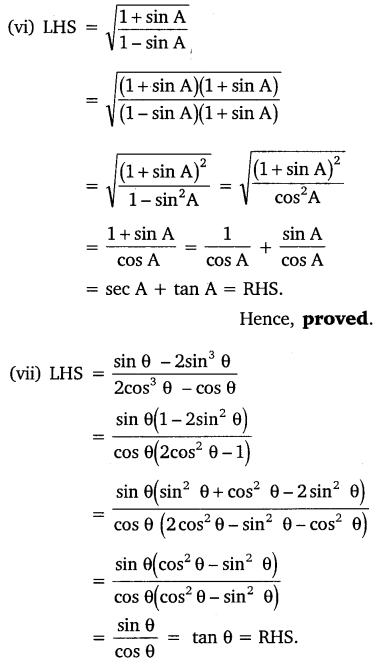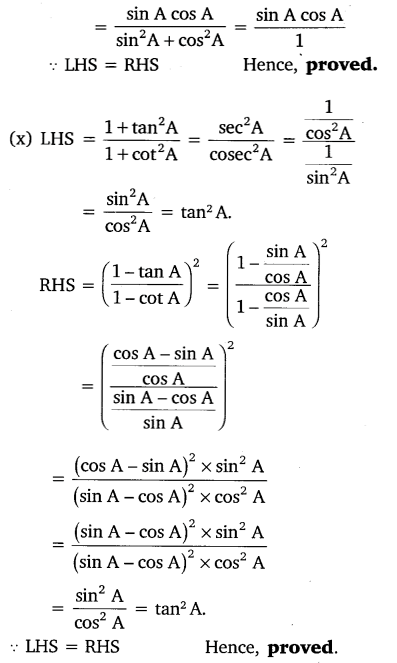### NCERT Solutions for Class 10 Maths Chapter 8 Introduction to Trigonometry (Hindi Medium) Ex 8.4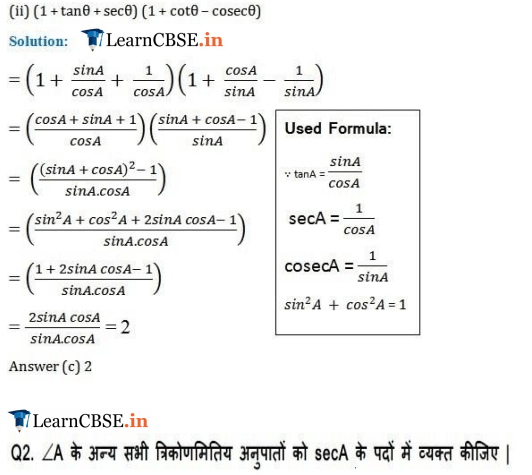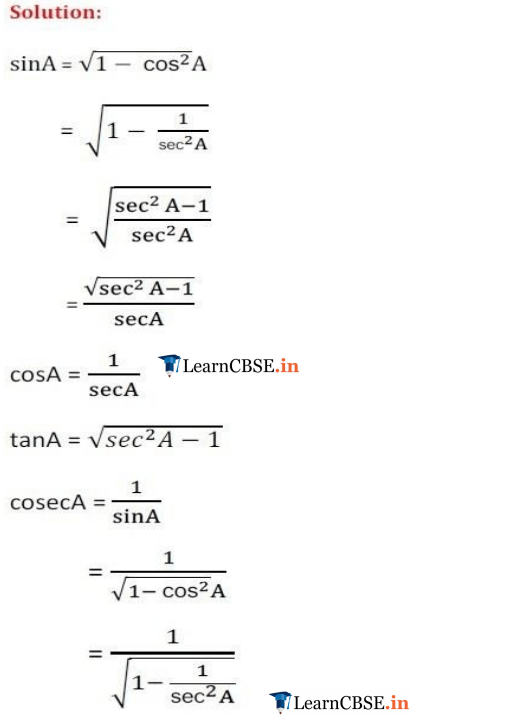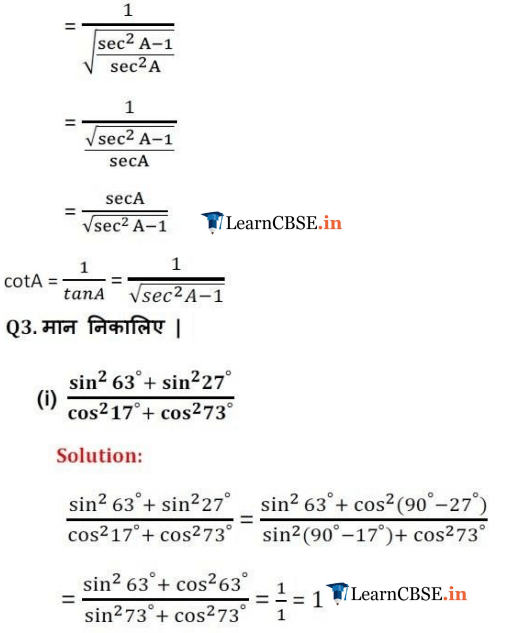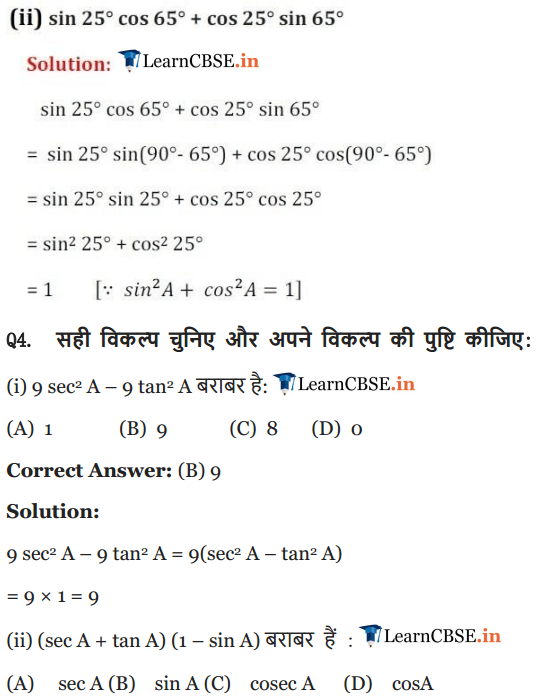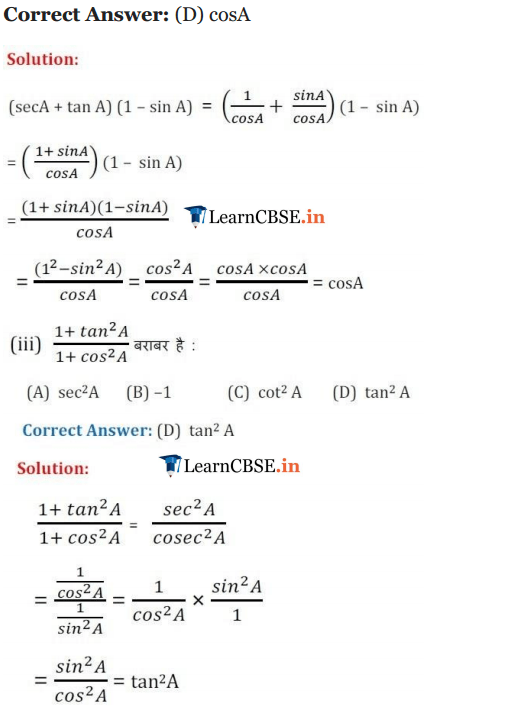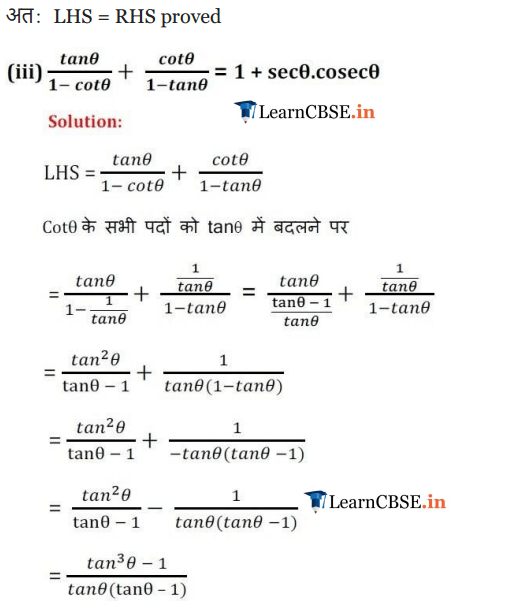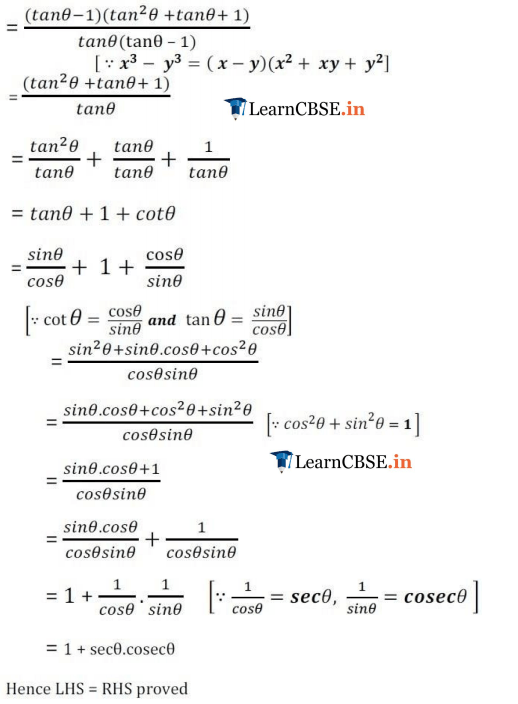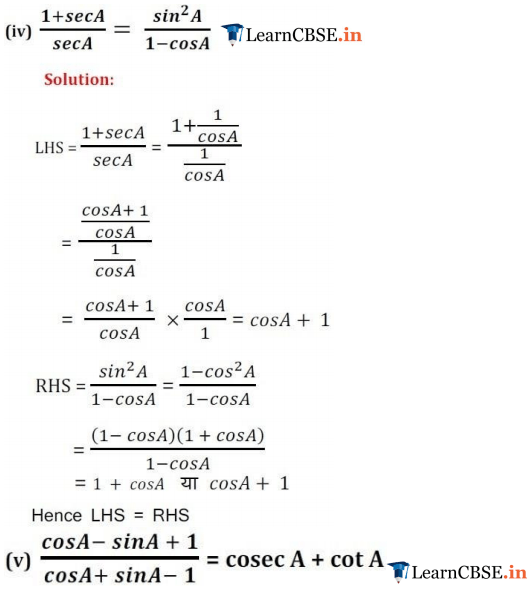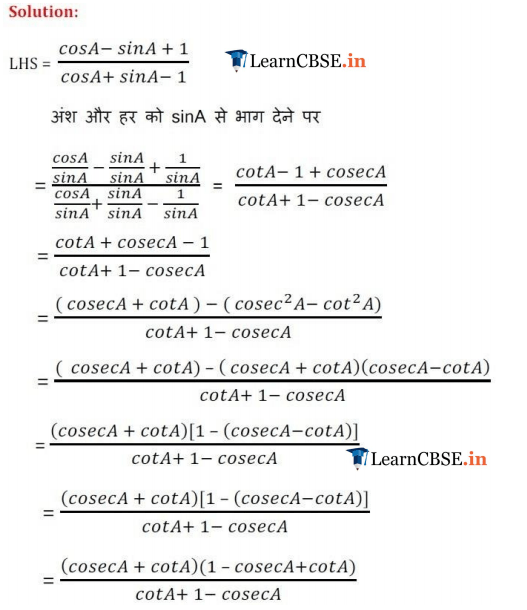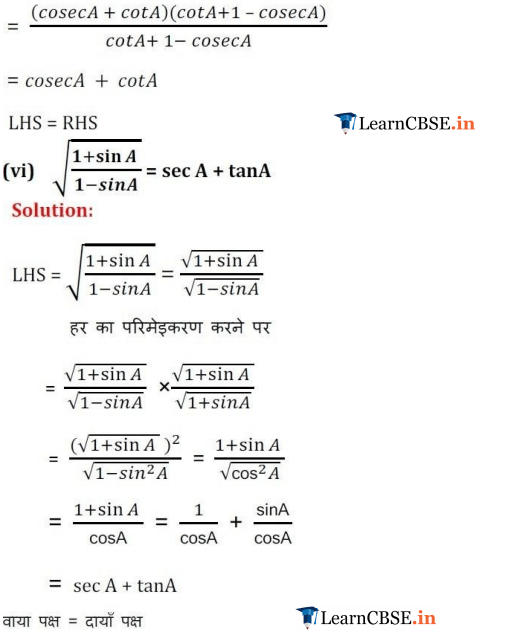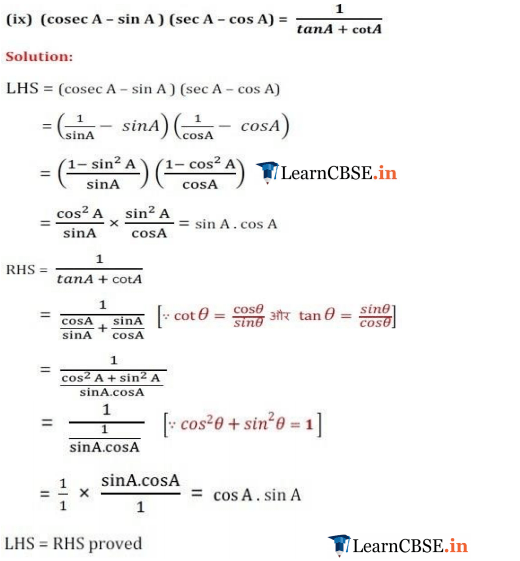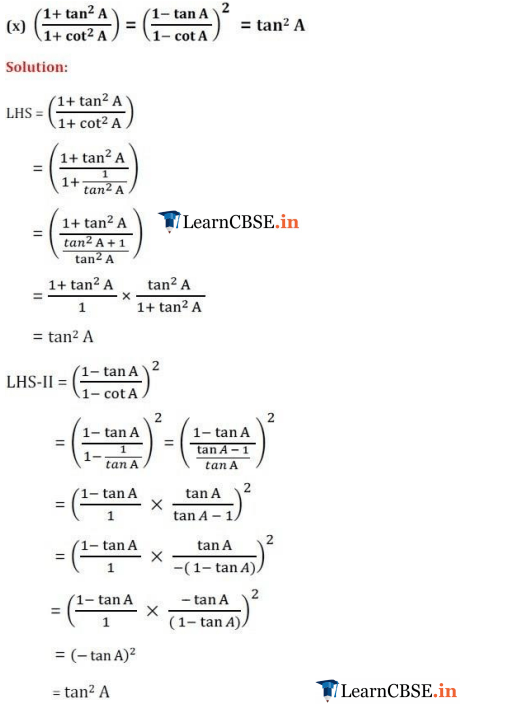We hope the NCERT Solutions for Class 10 Maths Chapter 8 Introduction to Trigonometry Ex 8.4, help you. If you have any query regarding NCERT Solutions for Class 10 Maths Chapter 8 Introduction to Trigonometry Exercise 8.4, drop a comment below and we will get back to you at the earliest.